## Building a Localized Single-Particle Wave Packet

We now have a wave function for a free particle with a definite momentum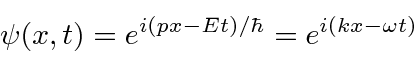where the wave number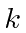is defined byand the angular frequencysatisfies. It is not localized sinceeverywhere.

We would like a state which is localized and normalized to one particle.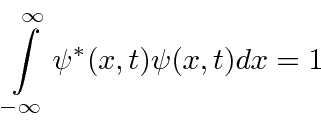To make a wave packet which is localized in space, we must add components of different wave number. Recall that we can use a Fourier Series to compose any function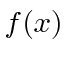when we limit the range to. We do not want to limit our states in, so we will take the limit that. In that limit, every wave number is allowed so the sum turns into an integral. The result is the very closely related Fourier Transformwith coefficients which are computable,The normalizations ofandare the same (with this symmetric form) and both can represent probability amplitudes.We understandas a wave packet made up of definite momentum terms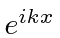. The coefficient of each term is. The probability for a particle to be found in a region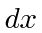around some value ofis. The probability for a particle to have wave number in regionaround some value ofis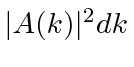. (Remember thatso the momentum distribution is very closely related. We work withfor a while for economy of notation.)

Jim Branson 2013-04-22